# Locally free sheaf

(diff) ← Older revision | Latest revision (diff) | Newer revision → (diff)
A sheaf of modules that is locally isomorphic to the direct sum of several copies of the structure sheaf. More precisely, let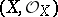be a ringed space. A sheaf of modulesover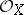is said to be locally free if for every pointthere is an open neighbourhood,, such that the restriction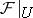oftois a free sheaf of modules over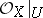, that is, it is isomorphic to the direct sum of a setof copies of the structure sheaf. Ifis connected and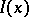is finite, for example consisting ofelements, thendoes not depend on the pointand is called the rank of the locally free sheaf. Letbe a vector bundle of rankonand letbe the sheaf of germs of its sections. Then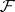is a locally free sheaf of rank. Conversely, for every locally free sheafof rankthere is a vector bundleof rankonsuch thatis the sheaf of germs of its sections (see , ); hence there is a natural one-to-one correspondence between the isomorphy classes of locally free sheaves of rankand the isomorphy classes of vector bundles of rankon.
Example. Letbe a smooth connected algebraic variety of dimension. Then the sheaf of regular differential formsis a locally free sheaf of rank.
Let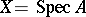, a connected affine scheme, be the spectrum of the commutative ring(cf. Spectrum of a ring), letbe a locally free sheaf of rankand let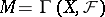be the-module of its global sections. Then the-moduleis projective and the mappingestablishes a one-to-one correspondence between the set of classes (up to isomorphisms) of locally free sheaves of rankand the set of classes (up to isomorphisms) of projective-modules of rank(see ).